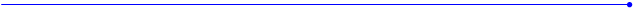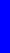The Nealon Equationy  =  (A*sin(B*x+C) + D*sin(E*x+F) + G*sin(H*x+I) + J*sin(K*x+L)) *  (M + N*x + O*x^2 + P*x^3 + Q*x^4) * atan(R*x) * atan(S*(BL-x))Benedetto Arch Top
 (A*sin(B*x+C) + D*sin(E*x+F) + G*sin(H*x+I) + J*sin(K*x+L) + M*sin(N*x+O) +P*sin(Q*x+R)+AA*sin(BB*x+CC) + DD*sin(EE*x+FF)) * (S + T*x + U*x^2 + V*x^3 + W*x^4) * atan(Y*x) * atan(Z*(20.82-x))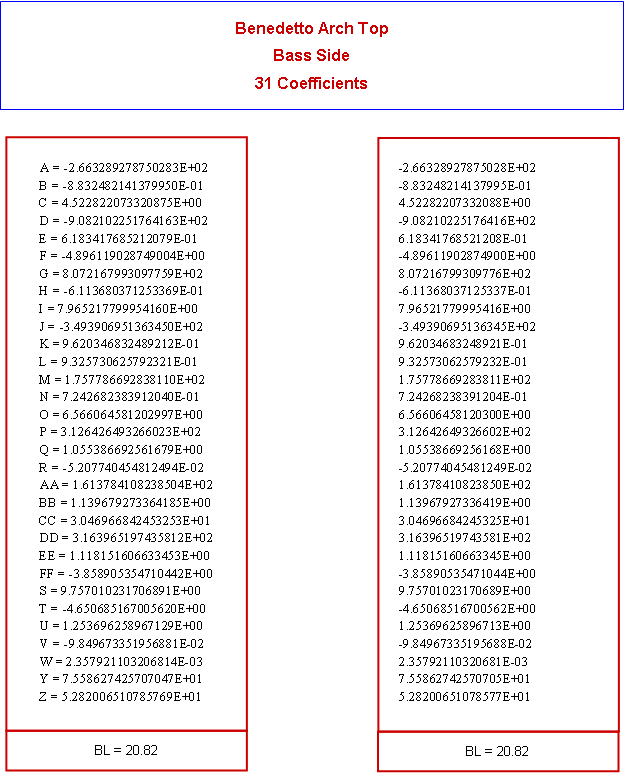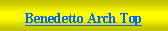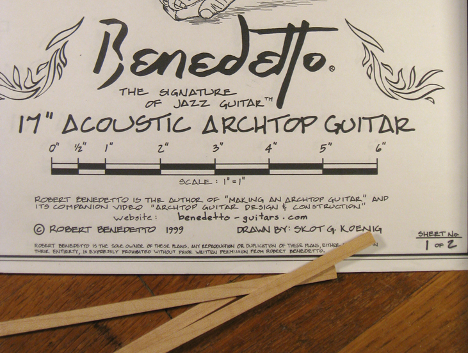Benedetto Guitars   http://benedettoguitars.com     Data from Blueprint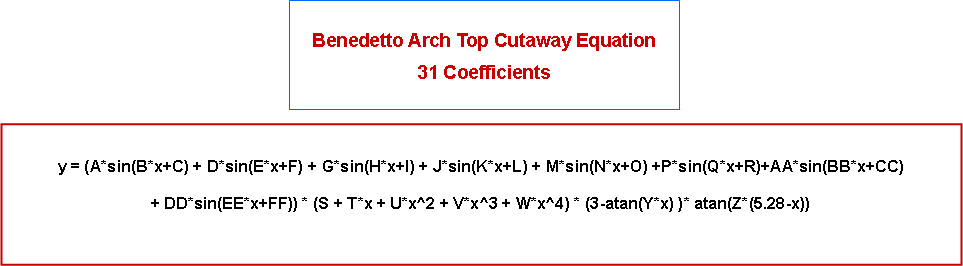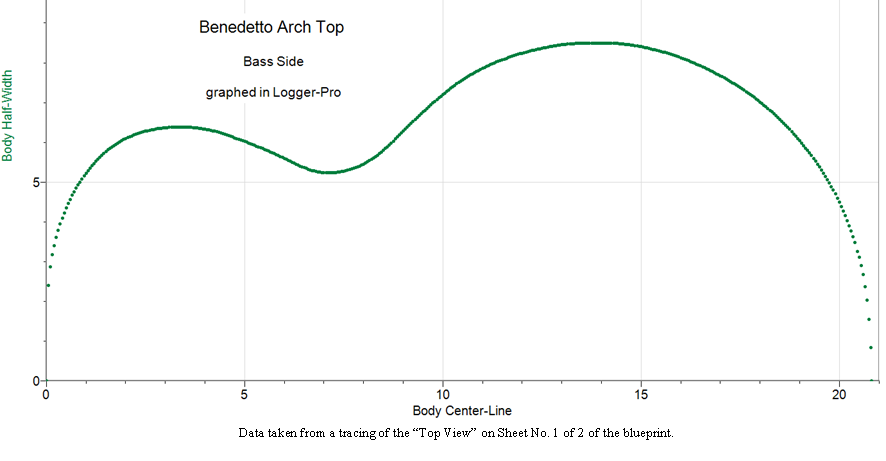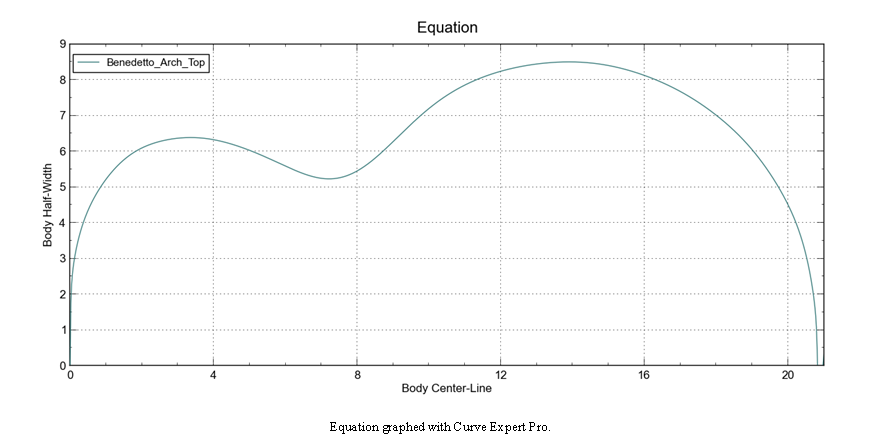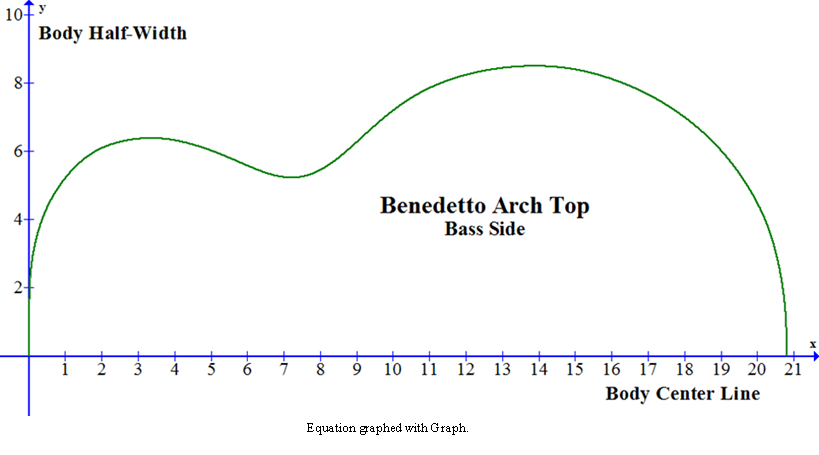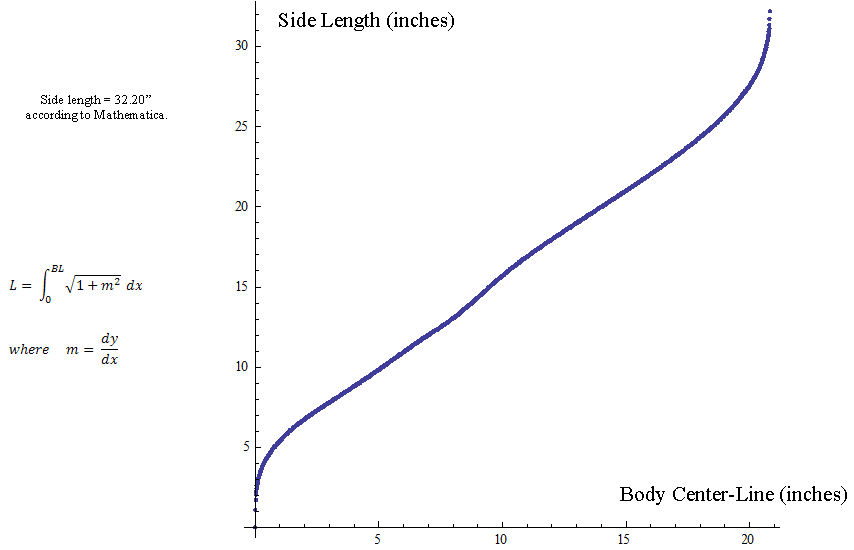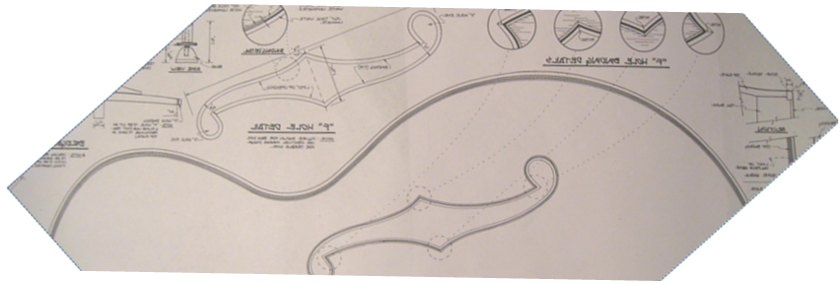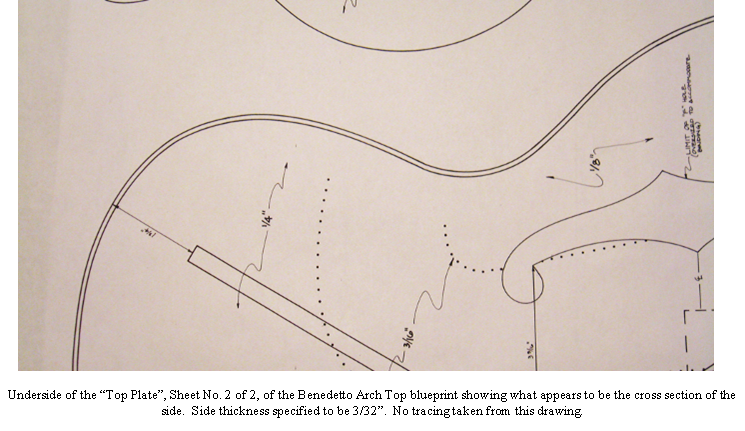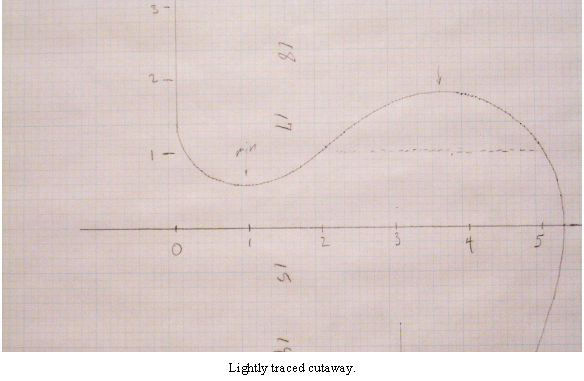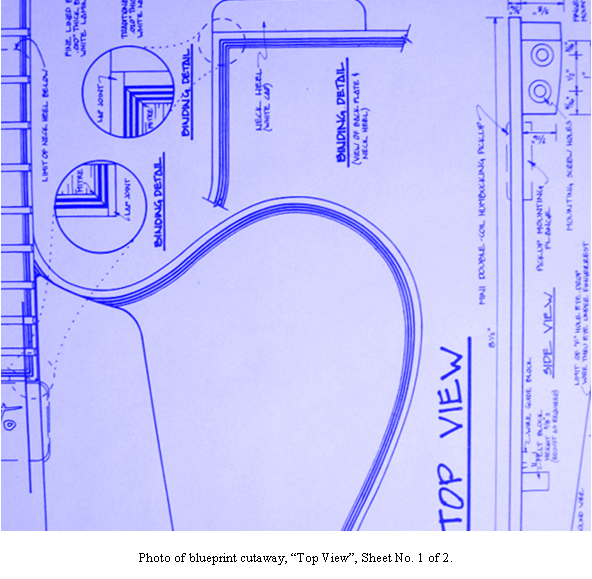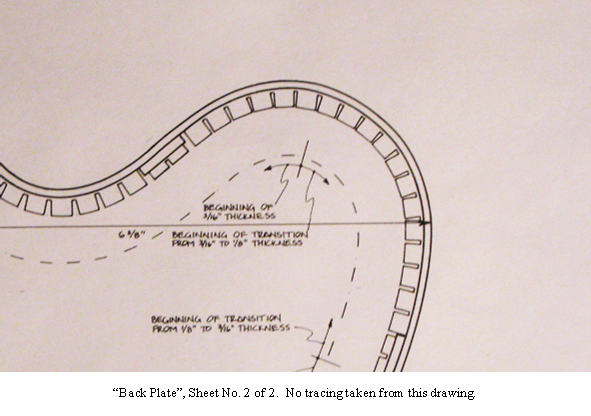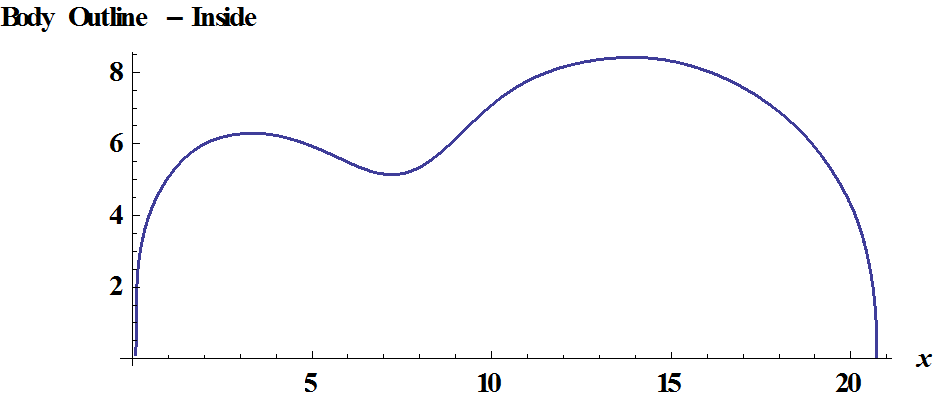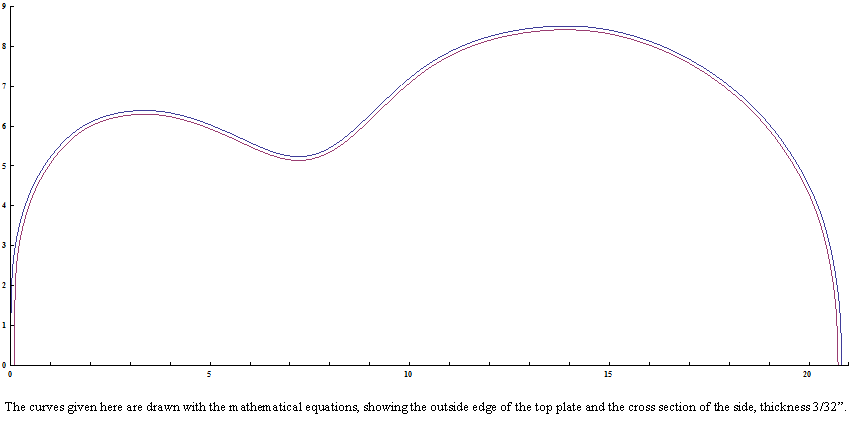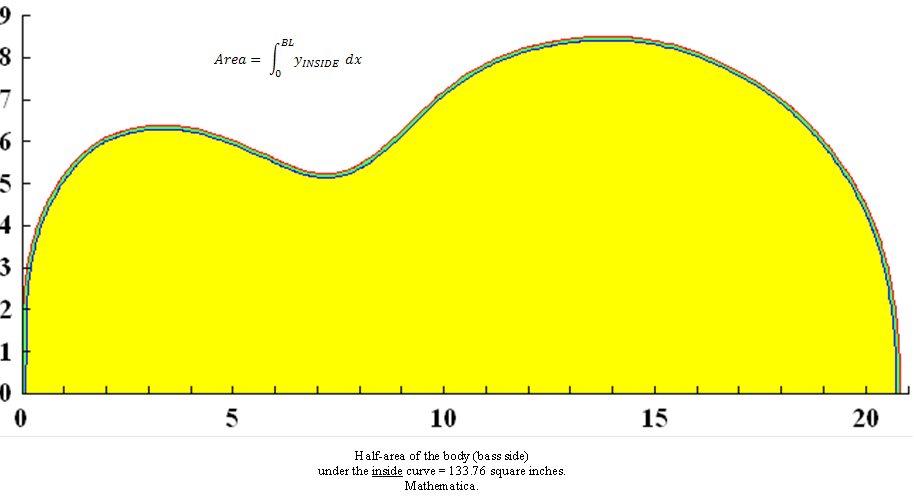In making the tracings of the bass side and the cutaway of this guitar, I followed the blueprint quite closely – quite.  Since the outline equation has so many coefficients, the mathematical curve which fits the tracing is flexible enough to follow along very precisely -  very.   Previously, I have correctly attributed any flat spots, or “lumpiness” in the outline curve to my imprecise tracing of a perfectly smooth pattern.  This lumpiness I refer to here is simply an aesthetic judgment of the smoothness of the curve to my eye.  In general, the guitar patterns I use to fit the equation are either the instruments themselves, a body mold, or blueprints.  These outlines are not my design (except the resonator) and the blueprints are not my drawings.  Up to this point I have not noticed any lumpiness in these original patterns.   This is the first outline of which I have noticed the following: lumpiness in the curve for this instrument is in the blueprint; my curve dutifully follows.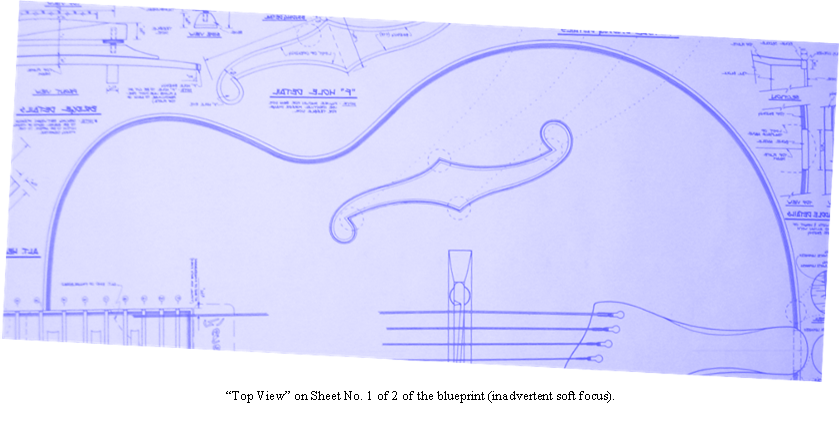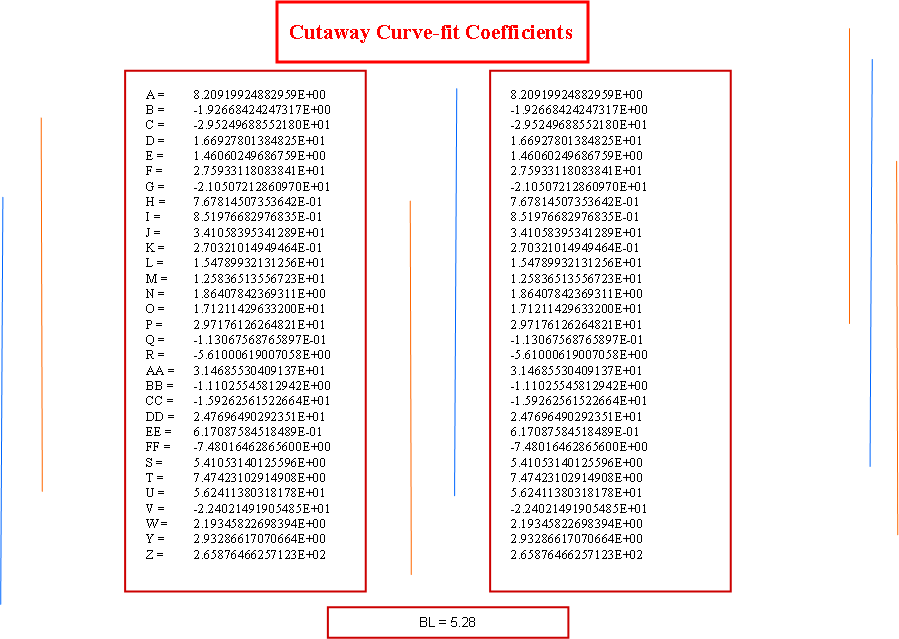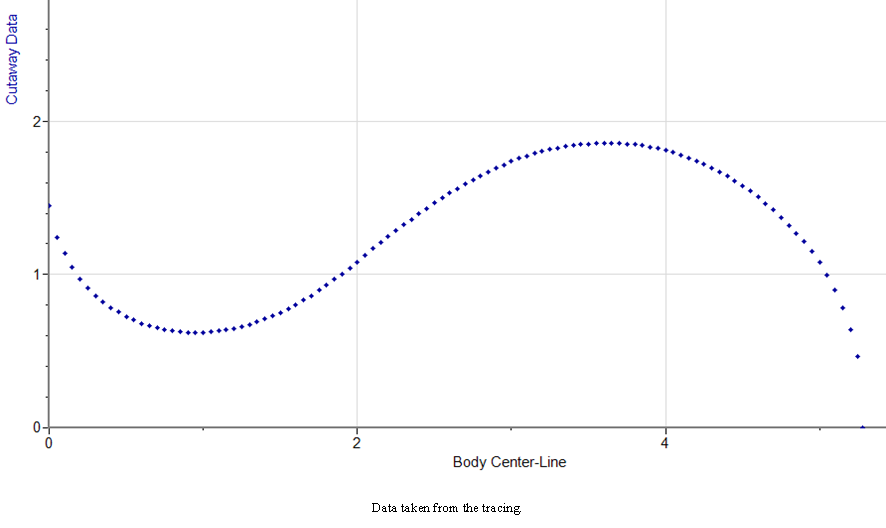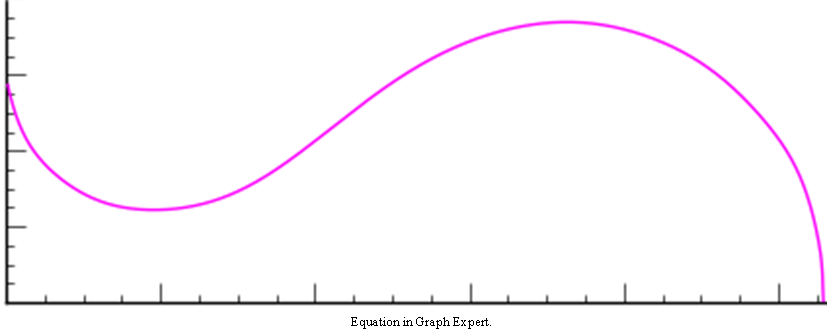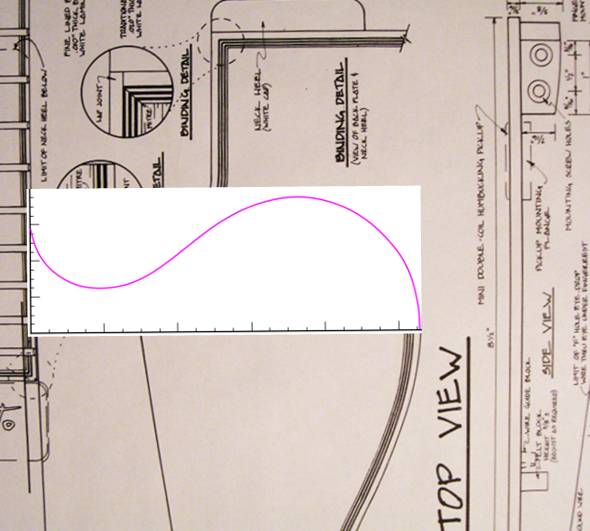In Mathematica: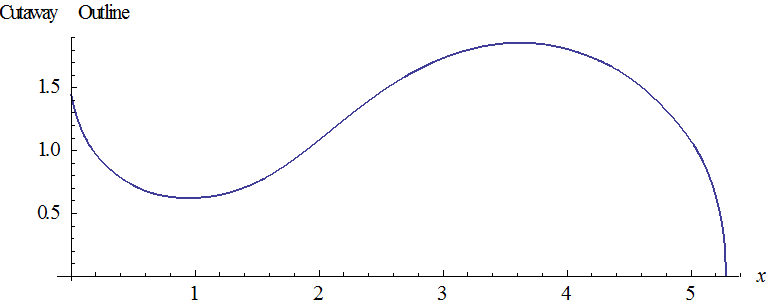Graphing artifact in Mathematica.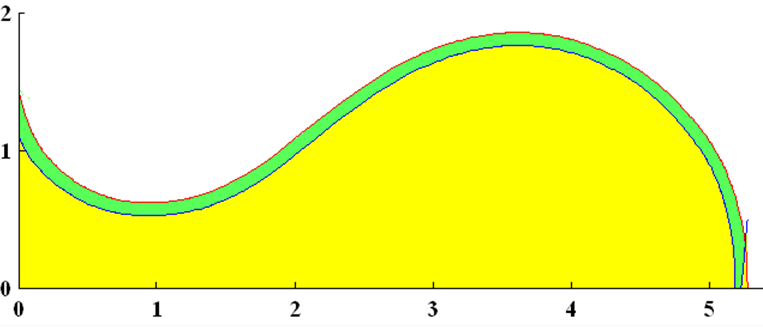Inside and Outside Edges of the Side at the Cutaway Side Thickness 3/32” Area of Cutaway Inside Curve (Yellow) = 5.88 square inches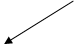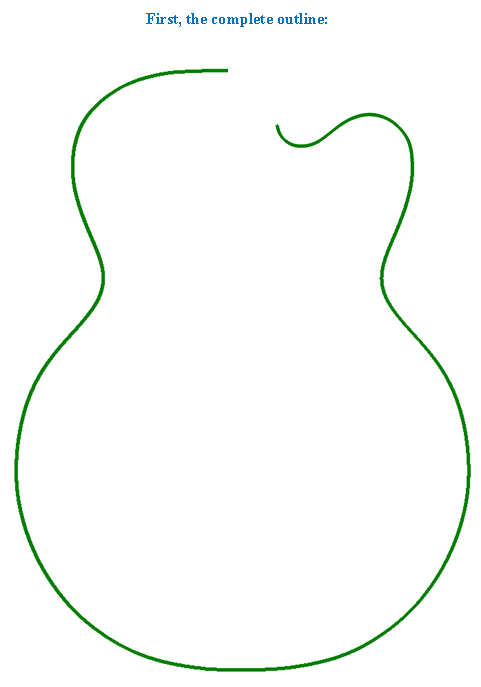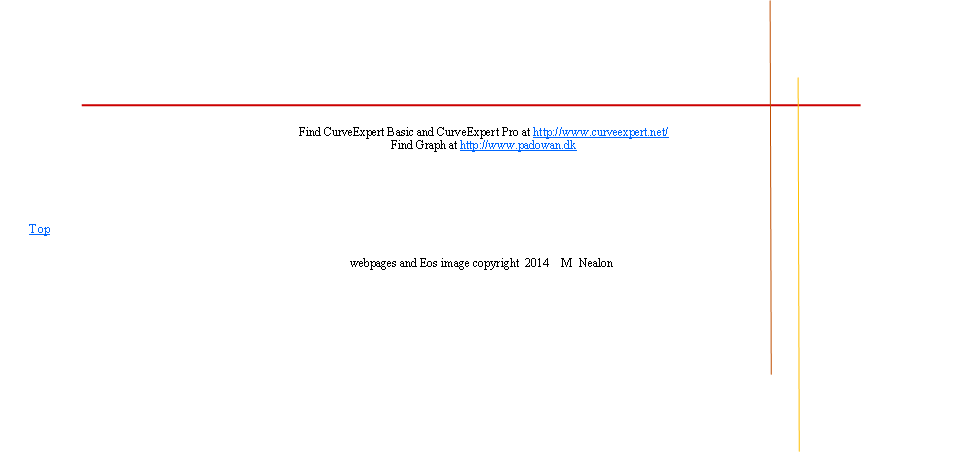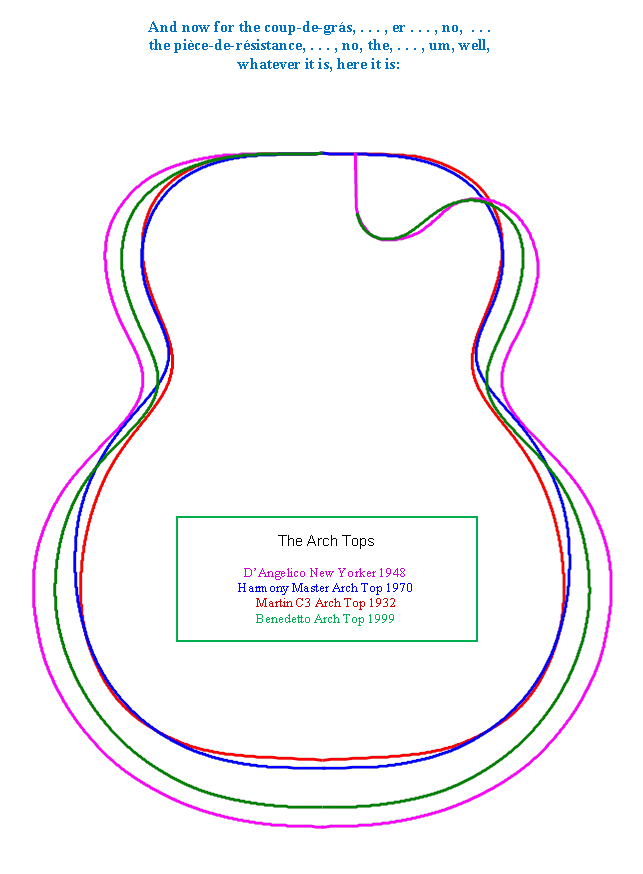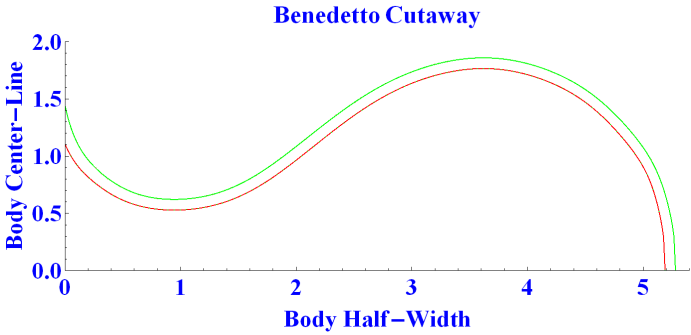Here it is removed, but I forgot to put in the shading.